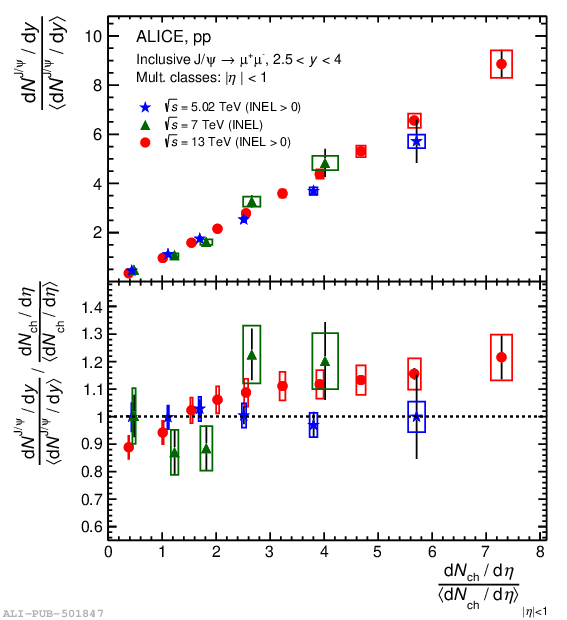# Figure 4

 Relative J/$\psi$ yield as a function of the relative charged-particle density measured at forward rapidity in \pp collisions at \s $=$ 5.02 (INEL$>$0), 7 (INEL) and 13 (INEL$>$0) TeV. The bottom panel shows the ratio of relative yield of $\rm{J}/\psi$ to relative charged-particle density. The vertical bars and the boxes represent the statistical and the systematic uncertainties, respectively.